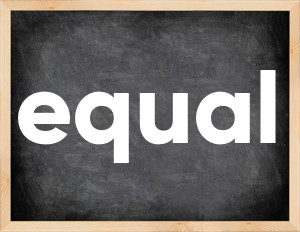# Equal past tenseThe English verb 'equal' is pronounced as [ˈiːkwəl].
Related to: regular verbs.
3 forms of verb equal: Infinitive (equal), Past Simple - (equaled), Past Participle - (equaled).

## Here are the past tense forms of the verb equal

👉 Forms of verb equal in future and past simple and past participle.
❓ What is the past tense of equal.

## Equal: Past, Present, and Participle Forms

Base Form Past Simple Past Participle
equal [ˈiːkwəl]

equaled [ˈiːkwəld]

equaled [ˈiːkwəld]

## What are the 2nd and 3rd forms of the verb equal?

🎓 What are the past simple, future simple, present perfect, past perfect, and future perfect forms of the base form (infinitive) 'equal'?

### Learn the three forms of the English verb 'equal'

• the first form (V1) is 'equal' used in present simple and future simple tenses.
• the second form (V2) is 'equaled' used in past simple tense.
• the third form (V3) is 'equaled' used in present perfect and past perfect tenses.

## What are the past tense and past participle of equal?

The past tense and past participle of equal are: equal in past simple is equaled, and past participle is equaled.

### What is the past tense of equal?

The past tense of the verb "equal" is "equaled", and the past participle is "equaled".

### Verb Tenses

Past simple — equal in past simple equaled (V2).
Future simple — equal in future simple is equal (will + V1).
Present Perfect — equal in present perfect tense is equaled (have/has + V3).
Past Perfect — equal in past perfect tense is equaled (had + V3).

### equal regular or irregular verb?

👉 Is 'equal' a regular or irregular verb? The verb 'equal' is regular verb.

## Examples of Verb equal in Sentences

•   John could not be equal to him in terms of discipline. (Past Simple)
•   In the midst of the war their production equaled the enemy. (Past Simple)
•   Three plus two equals five. (Present Simple)
•   His record has never been equaled or bettered. (Present Perfect)
•   He fought with a strenght that I have never seen equaled. (Present Perfect)
•   I think that my car equals yours in speed. (Present Simple)
•   Was he equal to the task? (Past Simple)
•   Let's see if you can equal that! (Present Simple)
•   No one can equal him in football. (Present Simple)
•   All people are equal before the law. (Present Simple)

Along with equal, words are popular point out and list.

Verbs by letter: , , , , , , , , , , , , , , , , , , , , , , , , .